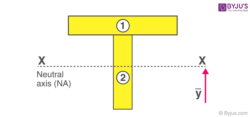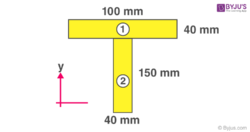# Moment of Inertia of T Section

Moment of Inertia is the quantity that expresses an object’s resistance to change its state of rotational motion. The moment of inertia of a T section is calculated by considering it as 2 rectangular segments. The moment of inertia is separately calculated for each segment and put in the formula to find the total moment of inertia.

## How to Find Moment of inertia of “T” section

The following steps should be followed to find the moment of inertia of the T section.Step 1: The beam sections should be segmented into parts

The T beam section should be divided into smaller sections. The moment of inertia must be calculated for the smaller segments. Here the section is divided into two rectangular segments.

Step 2: Mark the neutral axis

The neutral axis is the horizontal line passing through the centre of mass. The centre of mass is calculated using the following formula

Yc = ΣYiAi/ΣAi

Yi is the centre of mass of the individual rectangle

Ai is the area of the individual rectangle

Step 3: Calculating the Moment of Inertia

Using the parallel axis theorem the total momentum is calculated as

$I_{total}=\sum (\bar{I_{i}}+A_{i}d_{i}^{2})$

where,

$(\bar{I_{i}})$ is the inertia of the individual segment about its own centroid axis.

Ai is the individual segment

di is the vertical distance from the centroid of the segment.

The moment of inertia of each of the rectangular parts must be calculated. The moment of inertia equation of a rectangle about its centroid axis is

$\bar{I} = \frac{1}{12}bh^{3}$

Where,

b is the base of the rectangle

h is the height of the rectangle

## ExampleLet us understand the above equation with an example.

Finding the centre of mass of the T section

Yc = ΣYiAi/ΣAi

={[(150 + (40/2))(40 x 100)] + [(150/2) + (150 x40)]} / {[(40x 100) + (150 x40)]

=[(170 x 4000) + (75 x 600)]/ [4000 + 6000]

= 113 mm

Moment of inertia of T section, $I_{total}=\sum (\bar{I_{i}}+A_{i}d_{i}^{2})$

Where Ii is the moment of inertia of the individual segments

Ai is the area of the individual segments

di is the vertical distance from the centroid of the segment

The moment of inertia equation of a rectangle about its centroid axis is given by

$\bar{I} = \frac{1}{12}bh^{3}$

Where,

b is the base of the rectangle

h is the height of the rectangle

$(\bar{I_{1}})$ = (1/12)(100)(40)3

= 533,333 mm4

$(\bar{I_{2}})$ = (1/12)(40)(150)3

= 1.125 x 107 mm4

$I_{total}=\sum (\bar{I_{i}}+A_{i}d_{i}^{2})$ = [(533,333 + 4000(170 – 113)2] + [(1.125 x 107) + 6000(113 – 75)2]

Itotal = 3.3443 x 107 mm4